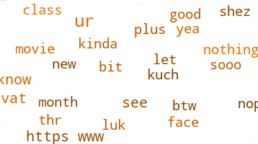Related Articles
Converting WhatsApp chat data into a Word Cloud using Python
• Last Updated : 02 Sep, 2020

Let us see how to create a word cloud using a WhatsApp chat file.

Convert the WhatsApp chat file from .txt format to .csv file. This can be done using Pandas. Create a DataFrame which read the .txt file. The .txt file had no columns like it is in an .csv file.

Then, split the data into columns by separating them and giving each column a name. The first line of the dataset in the chat file consists of encryption details which is not required here. Then, name the remaining 2 parts as Date and Convo, both parts of which are separated by a comma i.e. “,”.

## Python3

 `df ``=` `df.drop(``0``)``df.columns ``=` `[``'Date'``, ``'Convo'``]`

Now, separate the Convo data set into columns “Time” and “Content”, both of which are separated by a hyphen i.e. “-“. The data in Convo column is made into a data frame Chat.

## Python3

 `Chat ``=` `df[``"Convo"``].``str``.split(``"-"``, n ``=` `1``, ``                             ``expand ``=` `True``)``df[``'Time'``] ``=` `Chat[``0``]``df[``'Content'``] ``=` `Chat[``1``]`

The Content column is created into another data set Chat1, to divide it further into 2 columns, ”User” and “Message”, both of which are separated by a colon i.e. “:”.

## Python3

 `Chat1 ``=` `df[``"Content"``].``str``.split(``":"``, n ``=` `1``, ``                                ``expand ``=` `True``)``df[``'User'``] ``=` `Chat1[``0``]``df[``'Message'``] ``=` `Chat1[``1``]`

Now, dropping the Convo column and converting the Message column into lower case. All column media omitted cells and deleted messages are replaced by the string “Media Shared” and “DeletedMsg”.

## Python3

 `df ``=` `df.drop(columns ``=` `[``'Convo'``])``df[[``'Message'``] ``=` `df[``'Message'``].``str``.lower()``df[``'Message'``] ``=` `df[``'Message'``].``str``.replace(``''``, ``                                          ``'Media Shared'``)``df[``'Message'``] ``=` `df[``'Message'``].``str``.replace(``'this message was deleted'``, ``                                          ``'DeletedMsg'``)`

Finally, the data frame is converted to a .csv file named “new_csv.csv”.

## Python3

 `df.to_csv(``"new_csv.csv"``, index ``=` `False``)`

Now we have to make a word cloud from this CSV file. For this, we need word cloud and matplotlib.plyplot  packages. Use the new_csv.csv file to read data from it and create a data frame. Create a set of Stopwords and a variable to store all data generated from the WordCloud function. Extracte the data from the Message column containing all the chat texts and converted it into lowercased string.

## Python3

 `# importing the modules``import` `matplotlib.plyplot as mpl``from` `worcloud ``import` `WordCloud,STOPWORDS`` ` `# reading the csv file as a DataFrame``df1 ``=` `pd.read_csv(``"new_csv.csv"``)`` ` `# defining the stop words``stopwords ``=` `set``(STOPWORDS)``words``=``''.join(df1.Message.astype(``str``)).lower()`` ` `# making the word cloud``wordcloud ``=` `WordCloud(stopwords ``=` `stopwords, ``                      ``min_font_size ``=` `10``,``                      ``background_color ``=` `'white'``, ``                      ``width ``=` `800``,``                      ``height ``=` `800``,``                      ``color_func ``=` `random_color_func).generate(words)`

Here, function “random_color_func” which is used to render random orangish colors to the words. This is done by changing the values in the hsl (hue, saturation, luminance) values.

## Python3

 `def` `random_color_func(word ``=` `None``, ``                      ``font_size ``=` `None``, ``                      ``position ``=` `None``,  ``                      ``orientation ``=` `None``, ``                      ``font_path ``=` `None``, ``                      ``random_state ``=` `None``):``    ``h ``=` `int``(``360.0` `*` `21.0` `/` `255.0``)``    ``s ``=` `int``(``100.0` `*` `255.0` `/` `255.0``)``    ``l ``=` `int``(``100.0` `*` `float``(random_state.randint(``60``, ``120``)) ``/` `255.0``)`

Then use mpl to plot and visualize the words in wordcloud variable.

## Python3

 `mpl.figure(figsize ``=` `(``8``, ``8``), facecolor ``=` `None``)``mpl.imshow(wordcloud, interpolation ``=` `"bilinear"``)``mpl.axis(``"off"``)``mpl.tight_layout(pad ``=` `0``)``mpl.show()`The complete code is below:

## Python3

 `import` `pandas as pd``import` `matplotlib.plyplot as mpl``from` `worcloud ``import` `WordCloud, STOPWORDS`` ` `df ``=` `pd.read_csv(r``"WhatsAppChat.txt"``, ``                 ``header ``=` `None``, ``                 ``error_bad_lines ``=` `False``, ``                 ``encoding ``=` `'utf8'``)``df ``=` `df.drop(``0``)``df.columns ``=` `[``'Date'``, ``'Convo'``]``Chat ``=` `df[``"Convo"``].``str``.split(``"-"``, n ``=` `1``, ``                             ``expand ``=` `True``)``df[``'Time'``] ``=` `Chat[``0``]``df[``'Content'``] ``=` `Chat[``1``]``Chat1 ``=` `df[``"Content"``].``str``.split(``": "``, n ``=` `1``, ``                                ``expand``=``True``)``df[``'User'``] ``=` `Chat1[``0``]``df[``'Message'``] ``=` `Chat1[``1``]``df ``=` `df.drop(columns ``=` `[``'Convo'``])``df[``'Message'``] ``=` `df[``'Message'``].``str``.lower()``df[``'Message'``] ``=` `df[``'Message'``].``str``.replace(``'< media omitted >'``, ``'Media Shared'``)``df[``'Message'``] ``=` `df[``'Message'``].``str``.replace(``'this message was deleted'``, ``'DeletedMsg'``)``df.to_csv(``"new_csv.csv"``, index ``=` `False``)`` ` `def` `random_color_func(word ``=` `None``, ``                      ``font_size ``=` `None``, ``                      ``position ``=` `None``,  ``                      ``orientation ``=` `None``, ``                      ``font_path ``=` `None``, ``                      ``random_state ``=` `None``):``    ``h ``=` `int``(``360.0` `*` `21.0` `/` `255.0``)``    ``s ``=` `int``(``100.0` `*` `255.0` `/` `255.0``)``    ``l ``=` `int``(``100.0` `*` `float``(random_state.randint(``60``, ``120``)) ``/` `255.0``)`` ` `df1 ``=` `pd.read_csv(``"new_csv.csv"``)``stopwords ``=` `set``(STOPWORDS)``words ``=` `''.join(df1.Message.astype(``str``)).lower()`` ` `wordcloud ``=` `WordCloud(stopwords ``=` `stopwords, ``                      ``min_font_size ``=` `10``, ``                      ``background_color ``=` `'white'``, ``                      ``width ``=` `800``, ``                      ``height ``=` `800``, ``                      ``color_func ``=` `random_color_func).generate(words)`` ` `mpl.figure(figsize ``=` `(``8``, ``8``), facecolor ``=` `None``)``mpl.imshow(wordcloud, interpolation ``=` `"bilinear"``)``mpl.axis(``"off"``)``mpl.tight_layout(pad ``=` `0``)``mpl.show()`

Attention geek! Strengthen your foundations with the Python Programming Foundation Course and learn the basics.

To begin with, your interview preparations Enhance your Data Structures concepts with the Python DS Course. And to begin with your Machine Learning Journey, join the Machine Learning – Basic Level Course

My Personal Notes arrow_drop_up